Ch 6. Entropy Multimedia Engineering Thermodynamics Entropy Tds Relations EntropyChange IsentropicProcess IsentropicEfficiency EntropyBalance (1) EntropyBalance (2) ReversibleWork
 Chapter 1. Basics 2. Pure Substances 3. First Law 4. Energy Analysis 5. Second Law 6. Entropy 7. Exergy Analysis 8. Gas Power Cyc 9. Brayton Cycle 10. Rankine Cycle Appendix Basic Math Units Thermo Tables Search eBooks Dynamics Fluids Math Mechanics Statics Thermodynamics Author(s): Meirong Huang Kurt Gramoll ©Kurt GramollTHERMODYNAMICS - THEORY

Entropy Generation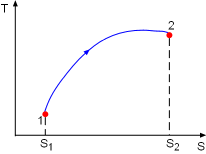Process 1-2 on T-s Diagram

Consider a system undergoing a process 1-2 as shown in the diagram.The increase of entropy principle states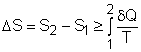The above equation can be expressed as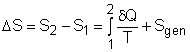Sgen > 0 irreversible process = 0 internally reversible process < 0 impossible
Non-negative Entropy Generation

where Sgen is called entropy generation. Sgen measures the effect of irreversibilities present within the system during a process. Its value depends on the nature of the process and not solely on the start and end states. Hence, it is not a property. Rewriting the above equation gives the expression of entropy generation as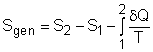According to the increase of entropy principle, entropy generation can only take a non-negative value.

Entropy Balance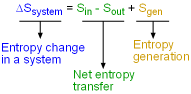Entropy Balance for a System

The entropy balance is an expression of the second law of thermodynamics that is particularly convenient for thermodynamic analysis. The increase of entropy principle states that the entropy can only be created, and it is expressed as

(Entropy change in a system)
=
(Total entropy entering the system)
-
(Total entropy leaving the system)
+
(Total entropy generation in the system)

or

ΔSsystem = Sin - Sout + Sgen

This expression is the entropy change of a system. It is applicable to any system undergoing any process. In unit-mass basis, it is

Δssystem = sin - sout + sgen

The first and second terms in the right hand side of the equation represent the entropy transfer between the system and its surroundings. The third term represents the entropy generation in the system.

Entropy Transfer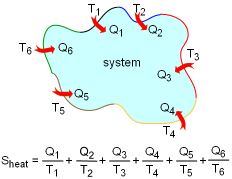Entropy Transfer by Heat with Different Boundary Temperatures

Entropy can be transferred to or from a system by two mechanisms: heat transfer and mass flow. Note that no entropy is transferred by work.

Heat Transfer

Heat transfer to a system increases the entropy of the system, and heat transfer out of a system decreases the entropy of the system.

During a process 1-2 of a system as defined above, If heat transfer δQ happens at the system boundary which has an absolute temperature T, the entropy transfer associated with this heat transfer process is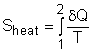If the entire boundary temperature remains as a constant T, the above equation can be simplified as

Sheat = Q/T

where
Q = total heat transfer during process 1-2

When the temperature T is not a constant, but a function of its location, the entropy change can be approximated as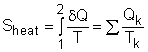where
Qk = heat transfer at boundary location k
Tk = temperature at boundary location k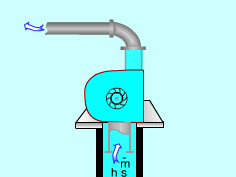Entropy Flows in or out with Mass

Mass Transfer

Mass contains entropy as well as energy. Mass m, which has a specific entropy s, contains a total entropy of ms. When a mass flows in a system, the entropy increase in the system is ms. In the same manner, mass m flowing out of a system will result in a decrease of entropy in amount of ms in the system.

Smass = ms

Entropy Balance for Closed Systems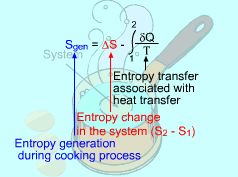Consider the Egg as
a Closed System

A closed system involves no mass transfer between the system and its surroundings, and thus its entropy transfer is only from heat transfer.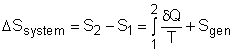If the closed system undergoes an adiabatic process, then no heat transfer occurs at the boundary. The entropy balance is reduced to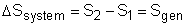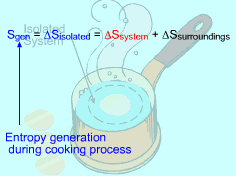Consider the Isolated System
Formed by the Egg and
the Surrounding Water

A system and its surroundings forms an isolated system. The entropy change of an isolated system formed by a closed system and its surroundings is

ΔSisolated = ΔSsystem + ΔSsurr = Sgen